### Home > MC1 > Chapter 3 > Lesson 3.4.4 > Problem3-128

3-128.
1. . Follow the order of operations (use circling the terms if it is helpful) to simplify the following expressions: Homework Help ✎

1. −7 + 4 ÷ (−2)

2. 14 ÷ (−7) + −8(2)

3. (8+ −3)(−5)

4. (4 − (−3)) + (5 ÷ (−5))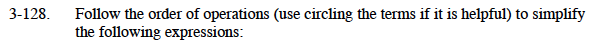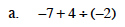If you need help with simplifying expressions, refer to problem 2-132. Try working it out on your own before checking the answer.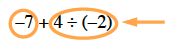−9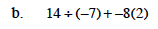Refer to part (a) if you are having trouble getting started. Remember to divide and multiply before adding or subtracting!

−18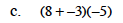This problem is similar to the previous two. Pay extra attention to parentheses!

Sophia thinks that the answer is 23, because multiplication should come before addition. Do you agree with her? Is there any reason that you might need to add first in this problem?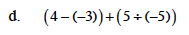This problem is similar to the previous three. Be careful to follow the order of operations as you calculate.